# Chemical Reactions Balancing Chemical Reactions Chemical Reactions Objectives

• Slides: 19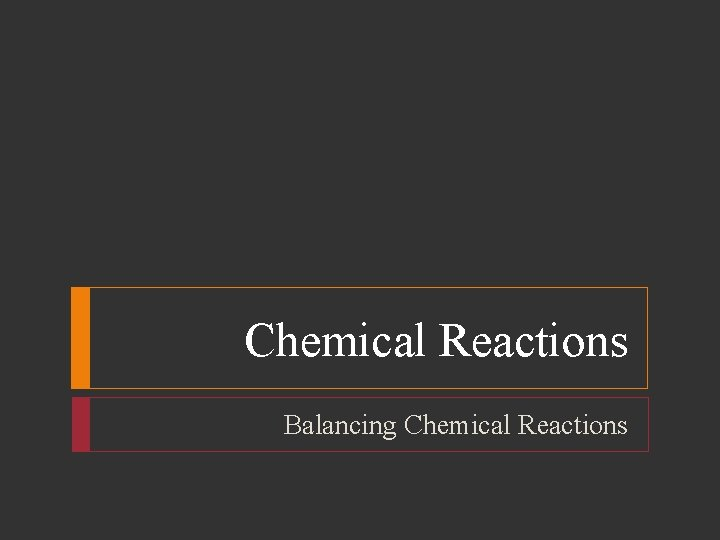Chemical Reactions Balancing Chemical ReactionsChemical Reactions Objectives List three observations that suggest that a chemical reaction has taken place List three requirements for a correctly written chemical equation Write a word equation and a formula equation for a given chemical reaction Balance a formula equation by inspectionChemical Reactions A chemical reaction is the process by which one or more substances are changed into one or more different substances In any chemical reaction, the original substances are known as the reactants and the resulting substances are known as the products According to the law of conservation of mass, the total mass of reactants must equal the total mass of products for any given chemical reaction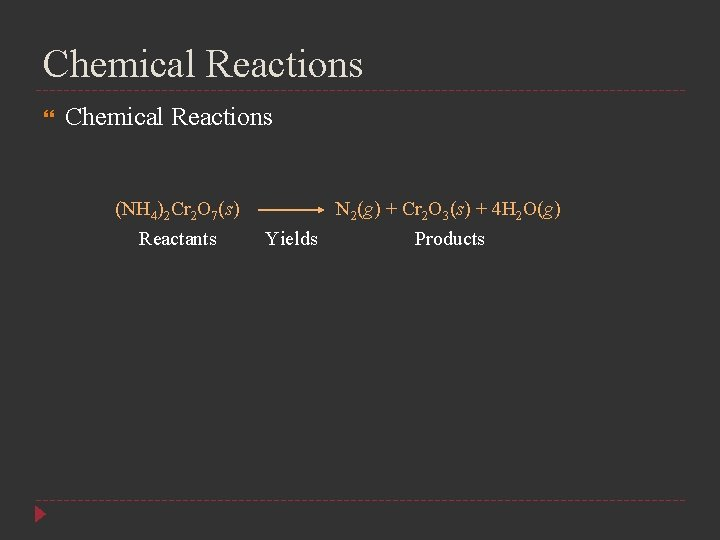Chemical Reactions (NH 4)2 Cr 2 O 7(s) Reactants N 2(g) + Cr 2 O 3(s) + 4 H 2 O(g) Yields ProductsChemical Reactions Indications of a Chemical Reaction Certain easily observed changes usually indicate that a chemical reaction has occurred Evolution of energy as heat and light Production of a gas Formation of a precipitate A solid that is produced as a result of a chemical reaction in solution and that separates from the solution is known as a precipitate Color changeChemical Reactions Chemical Equations The following requirements will aid you in writing and reading chemical equations correctly The equation must represent known facts The equation must contain the correct formulas for the reactants and products The law of conservation of mass must be satisfied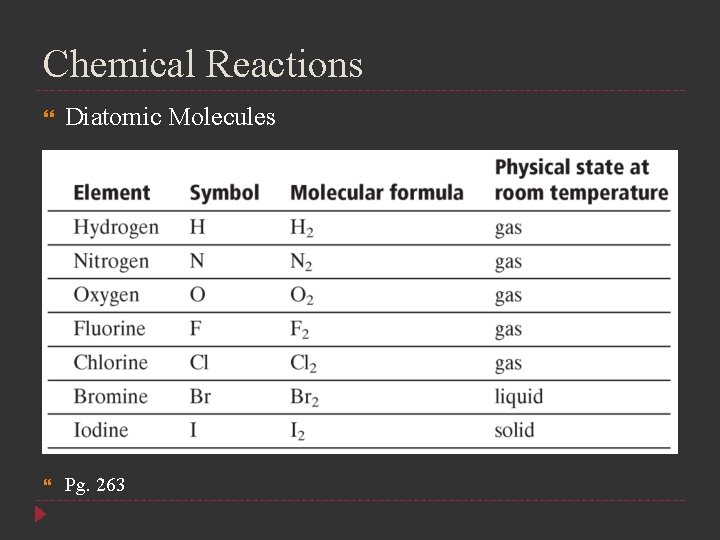Chemical Reactions Diatomic Molecules Pg. 263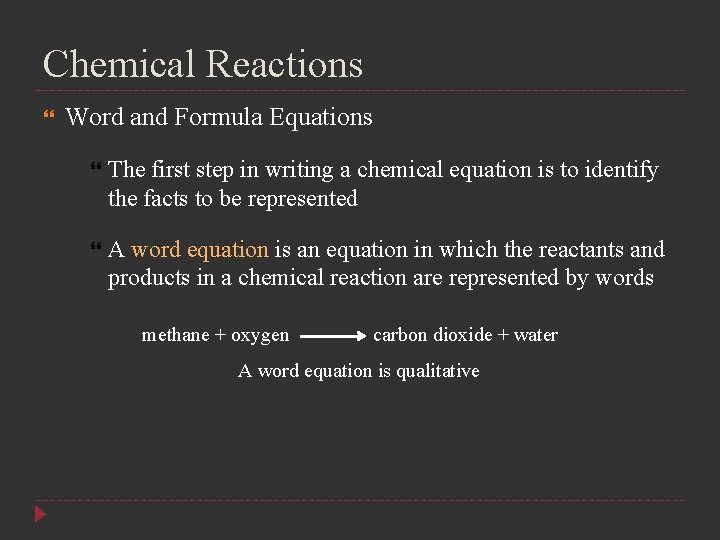Chemical Reactions Word and Formula Equations The first step in writing a chemical equation is to identify the facts to be represented A word equation is an equation in which the reactants and products in a chemical reaction are represented by words methane + oxygen carbon dioxide + water A word equation is qualitativeChemical Reactions Word and Formula Equations The next step in writing a correct chemical equation is to replace the names of the reactants and products with appropriate symbols and formulas A formula equation represents the reactants and products of a chemical reaction by their symbols or formulas CH 4(g) + O 2(g) CO 2(g) + H 2 O(g) not balanced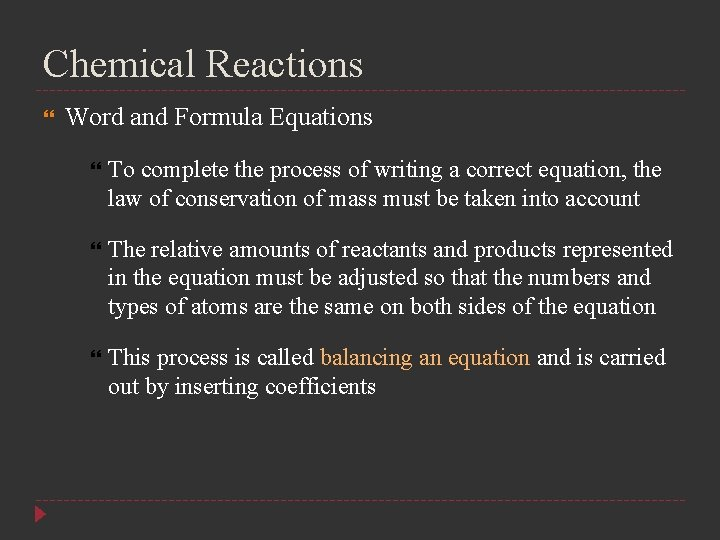Chemical Reactions Word and Formula Equations To complete the process of writing a correct equation, the law of conservation of mass must be taken into account The relative amounts of reactants and products represented in the equation must be adjusted so that the numbers and types of atoms are the same on both sides of the equation This process is called balancing an equation and is carried out by inserting coefficientsChemical Reactions Word and Formula Equations To balance the equation, begin by counting atoms of elements that are combined with atoms of other elements and that appear only once on each side of the equation CH 4(g) + O 2(g) CO 2(g) + 2 H 2 O(g) Partially Balanced CH 4(g) + 2 O 2(g) CO 2(g) + 2 H 2 O(g) Balanced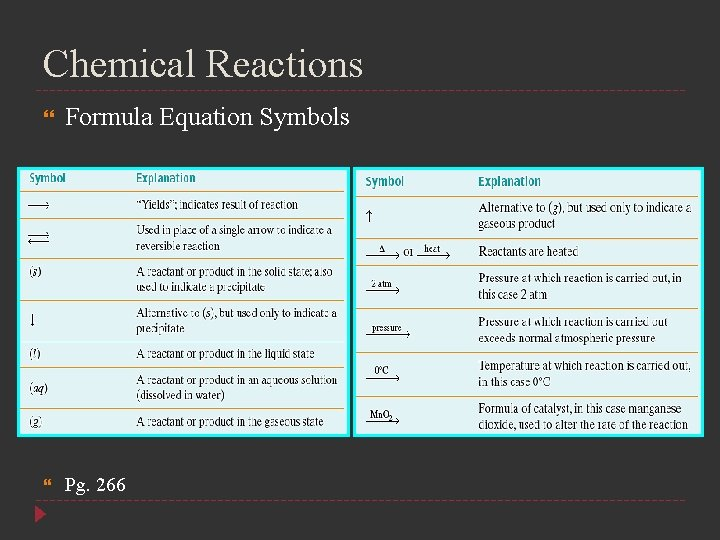Chemical Reactions Formula Equation Symbols Pg. 266Chemical Reactions Word and Formula Equations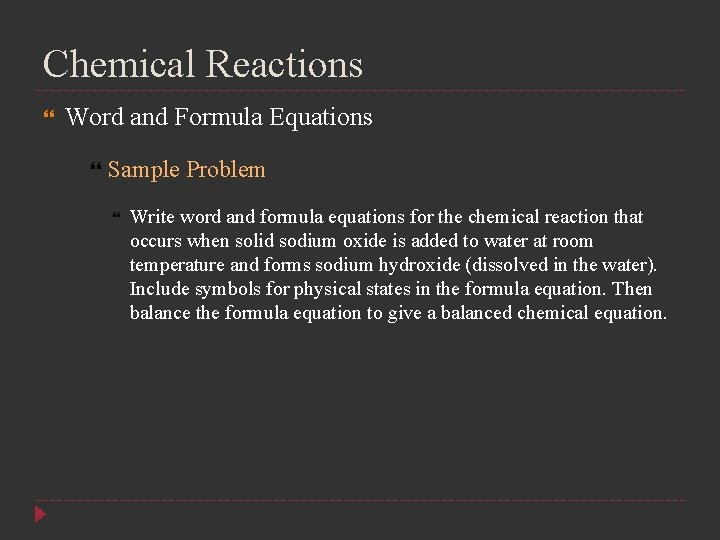Chemical Reactions Word and Formula Equations Sample Problem Write word and formula equations for the chemical reaction that occurs when solid sodium oxide is added to water at room temperature and forms sodium hydroxide (dissolved in the water). Include symbols for physical states in the formula equation. Then balance the formula equation to give a balanced chemical equation.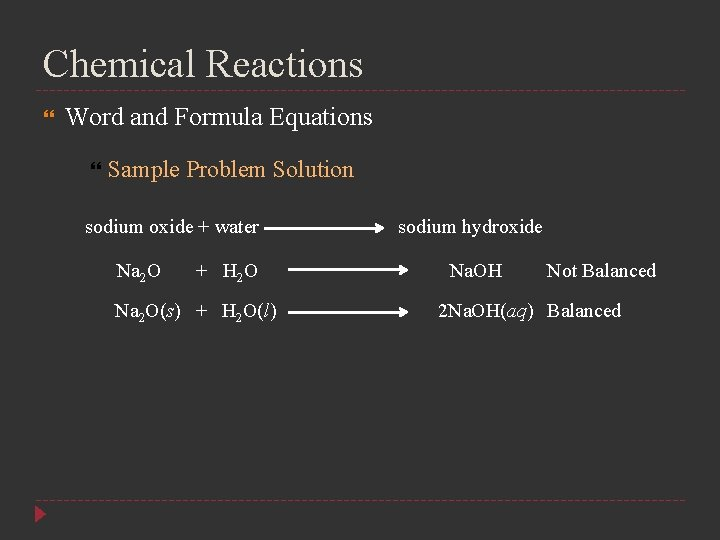Chemical Reactions Word and Formula Equations Sample Problem Solution sodium oxide + water Na 2 O + H 2 O Na 2 O(s) + H 2 O(l) sodium hydroxide Na. OH Not Balanced 2 Na. OH(aq) Balanced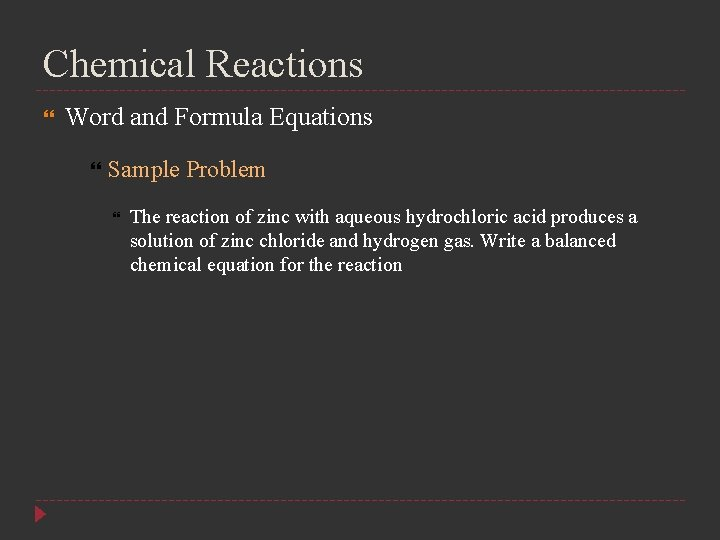Chemical Reactions Word and Formula Equations Sample Problem The reaction of zinc with aqueous hydrochloric acid produces a solution of zinc chloride and hydrogen gas. Write a balanced chemical equation for the reaction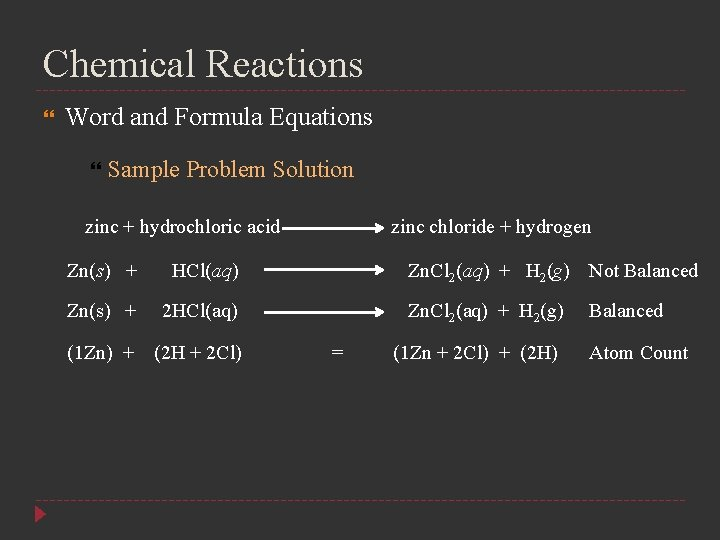Chemical Reactions Word and Formula Equations Sample Problem Solution zinc + hydrochloric acid Zn(s) + HCl(aq) Zn(s) + 2 HCl(aq) (1 Zn) + (2 H + 2 Cl) zinc chloride + hydrogen Zn. Cl 2(aq) + H 2(g) Not Balanced Zn. Cl 2(aq) + H 2(g) = (1 Zn + 2 Cl) + (2 H) Balanced Atom Count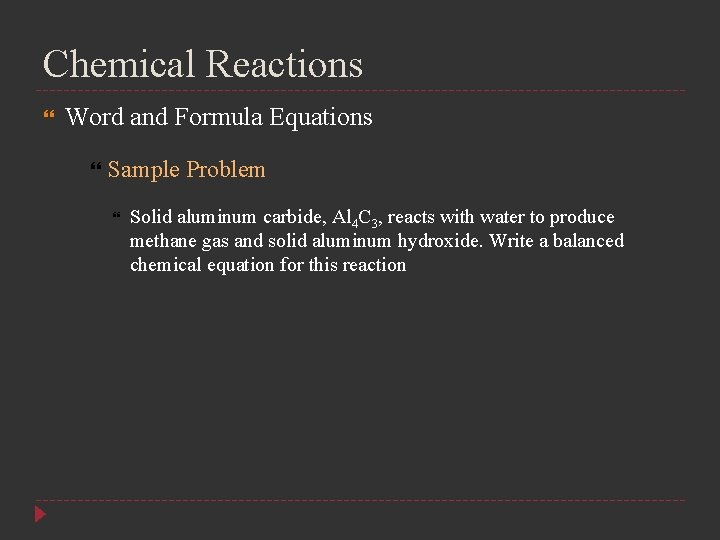Chemical Reactions Word and Formula Equations Sample Problem Solid aluminum carbide, Al 4 C 3, reacts with water to produce methane gas and solid aluminum hydroxide. Write a balanced chemical equation for this reactionChemical Reactions Word and Formula Equations Sample Problem Solution Al 4 C 3(s) + H 2 O(l) CH 4(g) + Al(OH)3(s) Al 4 C 3(s) + H 2 O(l) CH 4(g) + 4 Al(OH)3(s) Not Balanced Al 4 C 3(s) + H 2 O(l) 3 CH 4(g) + 4 Al(OH)3(s) Not Balanced Al 4 C 3(s) + 12 H 2 O(l) (4 Al + 3 C) + (24 H + 12 O) = Atom Count 3 CH 4(g) + 4 Al(OH)3(s) Balanced (3 C + 12 H) + (4 Al + 12 H + 12 O)